Function Repository Resource:

# RepeatingDecimalToRational

Find a rational number equivalent to a real number with repeating digits

Contributed by: Wolfram|Alpha Math Team
 ResourceFunction["RepeatingDecimalToRational"][x,n] returns a rational number that approximates x, assuming the last n digits of x repeat infinitely. ResourceFunction["RepeatingDecimalToRational"][x,{a,b,c,…}] returns a rational number that approximates x with the repeating digits {a,b,c,…} appended.

## Details and Options

ResourceFunction["RepeatingDecimalToRational"] expects a real number as its first argument.

## Examples

### Basic Examples (3)

Find a fractional representation for 0.333:

 In:=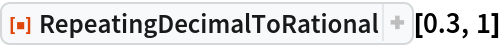Out=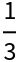Check the fractional representation of 1/7:

 In:=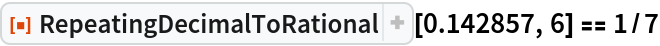Out=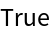Specify which digits are repeating:

 In:=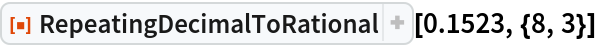Out=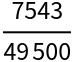Numericize that:

 In:=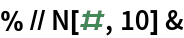Out=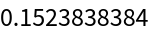## Publisher

Wolfram|Alpha Math Team

## Version History

• 2.0.0 – 23 March 2023
• 1.0.0 – 20 April 2020